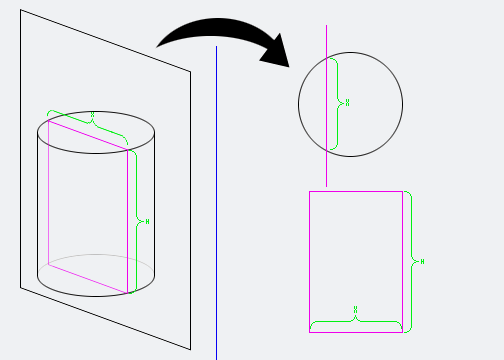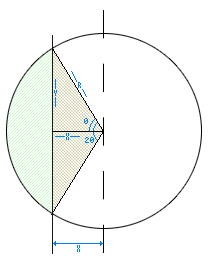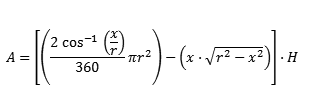## Recommended Posts

Imagine a a soda can (or any cylinder). If I had cut, arbitrarily, a chord, straight down (from the tab to the base)..., what would be the volume of that piece. Obviously, if it was cut directly in the middle, it'd just be half the total volume. I tried finding the volume of a sector. but that doesn't work for chords. For the sake of convenience, the chord will always be parallel to the radius for now.

I can't figure it out using calculus or geometry/trigonometry.

If you have trouble thinking about it..., think of a perfect circle with the center at the origin, then cutting a line parallel to the x axis . . . but it's arbitrarily placed.

I'd appreciate any help, thanks.

##### Share on other sites

There is no spoon Neo....

##### Share on other sites

There is no spoon Neo....

:(

##### Share on other sites

If you simplify to a circle rather than a cylinder then.

1. If you mark the cord, where it cross the the circumference draw lines into the center point.

2. Find the angle between the two lines which converge at the center point.

3. Work out the area of that section of the circle.

4. Now with those to lines you can form a triangle with the chord that cut it.

5. Find the area of this.

6. Minus the triangle area from the section of the circle area.

You now have the area of the circle cut away from the cord, which you could minus from the whole area to get the area of the circle without the cord.

Obvious for a cylinder you just need to put it back in 3D.

Hope this helps and is what you wanted.

##### Share on other sites

Would your calculation need to be for anything other than cylinders?

If it it just a cylinder, and the cutting plane is always perpendicular the calculation in very easy.

You just convert the Cylinder to a circle and the cutting plane to a line. You can then computer the two intersections find the distance between the two points and multiply by height.##### Share on other sites

Would your calculation need to be for anything other than cylinders?

If it it just a cylinder, and the cutting plane is always perpendicular the calculation in very easy.

You just convert the Cylinder to a circle and the cutting plane to a line. You can then computer the two intersections find the distance between the two points and multiply by height.Yes yes, this is a perfect representation. How, exactly do I find the volume of either piece (as divided by the pink line) without necessarily having the rest. I think I tried integrating infinitely many of those rectangles created by the cylinder (from the bottom to the top, as if it was lying on its side horizontally, not circle base to circle top) but my equation must have been wrong. If it was right, the answer would have been the same as $\pi$r^2*h, but it wasn't.

Stringwray's suggestions were helpful in finding the area of that chunk, but I couldn't figure out how to translate into 3D.

EDIT: Yes, only cylinders for now.

##### Share on other sites

Yes yes, this is a perfect representation. How, exactly do I find the volume of either piece (as divided by the pink line) without necessarily having the rest. I think I tried integrating infinitely many of those rectangles created by the cylinder (from the bottom to the top, as if it was lying on its side horizontally, not circle base to circle top) but my equation must have been wrong. If it was right, the answer would have been the same as $\pi$r^2*h, but it wasn't.

Stringwray's suggestions were helpful in finding the area of that chunk, but I couldn't figure out how to translate into 3D.

EDIT: Yes, only cylinders for now.

Well I was assuming that the chord would be parallel to the z-axis so that to make it into 3D you would just need to multiple by the z-axis difference value.

If you want it so that the chord is at an angle so that the area at the top and bottom is different. Then that needs some serious thought.

##### Share on other sites

What is your input viable? Distance of slicing plane from the center, the edge?

---------------

Here, i just threw together this equation.

x= distance from center of circle (0<x<r)

H= heightcalculations in DegreesA=[((2 arccos(x/r))/360 ⋅ πr^2 )-(x⋅√(r^2-x^2 )) ]⋅HA=[((2 arccos(x/r))/2π ⋅ πr^2 )-(x⋅√(r^2-x^2 )) ]⋅H

BTW the new math input panel in windows 7 is awesome

## Join the conversation

You can post now and register later. If you have an account, sign in now to post with your account.×   Pasted as rich text.   Paste as plain text instead

Only 75 emoji are allowed.

×   Your previous content has been restored.   Clear editor

×   You cannot paste images directly. Upload or insert images from URL.

×